### A Brief Guide to Engineering Financial Calculations: Depreciation

Depreciation is a non-cash expense that represents the need to replace assets eventually as they wear out. It is an accounting line item that tracks an estimate of how much asset value has been lost during the reporting period. Different types of assets have different rates at which they lose value. This decline in value is not always linear, because some assets lose value faster earlier in their life, and some assets hold most of their value early but then lose value faster later on. Some assets have residual value at the end of their useful life, called salvage value. Book value is whatever the value of the asset is estimated to be at that time (purchase price minus accumulated depreciation). Depreciation of intangible assets is also called amortization.

Key Assumptions

Unless otherwise stated, the fiscal year for a company is the calendar year.

The useful life of the asset is estimated to be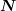years. The depreciation amount in the first year is adjusted if the asset is purchased in the middle of the year.

The Book Value for yearis,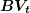and it can not be less than the Salvage Value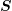.

Calculations

Straight-Line Method:

The depreciation amount for each full year is the same amount: the original value of the asset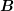minus the salvage valueall divided by the number of years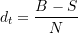The Book Value at the end of yearis the original book valueminus the sum of the accumulated depreciation amounts.

Sum-of-Years-Digits Method:
The depreciation amount is different for each year(wherestarts at 1 and goes to):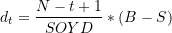The Book Value at the end of yearis the original book valueminus the sum of the accumulated depreciation amounts.

Declining Balance Method:
The depreciation amount in a given year depends on a depreciation rate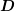multiplied by the Book Value for the previous year (which in the first year is the original value).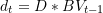where the Book Value at the end of yearis the original book valueminus the sum of the accumulated depreciation amounts: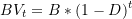thus we can directly write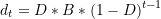Note that this method does not explicitly use an estimate of the useful life ofyears or salvage value, but the rule still applies thatcan not be less than.

Double Declining Balance Method:

This is a special case of the declining balance method, where a useful lifeis defined, from which the depreciation rate is set to be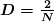Units of Production Method:

The depreciation amount in a given yeardepends on the actual production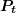and the total lifetime production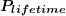over the useful life ofyears. The units of production will be in terms of the production method, such as tonnes of oilsand moved by a haul truck, number of widgets stamped out by a press, etc.

The Book Value at the end of yearis the original book valueminus the sum of the accumulated depreciation amounts: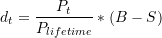For Assets Purchased Midway through a Year:

When an asset is purchased in the middle of a year, depreciation is prorated in that year. The calculation method is as follows:

1) Calculate the nominal annual depreciation for the first year using the chosen method (straight-line, SOYD, etc).

2) Book 1/12 of the nominal first-year depreciation amount per month on a straight-line basis for the remainder of the year, booking the first depreciation at the end of the month in which the asset was purchased. For example, a purchase in April entails nine depreciation amounts for the remainder of the year (end of April, end of May,…, end of December). Note that when an asset is purchased midyear, the nominal salvage valueis not actually achieved at the end of the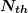year after the purchase.

Depreciation for Tax Purposes: Capital Cost Allowance

Capital cost allowance is calculated based on declining balance. When calculating capital cost allowance for year(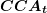) and the unclaimed capital allowance (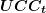), group assets by their taxation class and use the appropriate rate for each class. The sum of transactions for yearis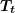; an asset transaction is positive for purchase of an asset & negative for a sale of an asset.  The CCA is not affected by when the assets are purchased during the tax year.

For each class of asset, the calculation procedure is:

The Book Value at the end of yearis the original book valueminus the sum of the accumulated depreciation amounts.

Historically, companies have most often used one of two methods:

straight-line, or double-declining balance.